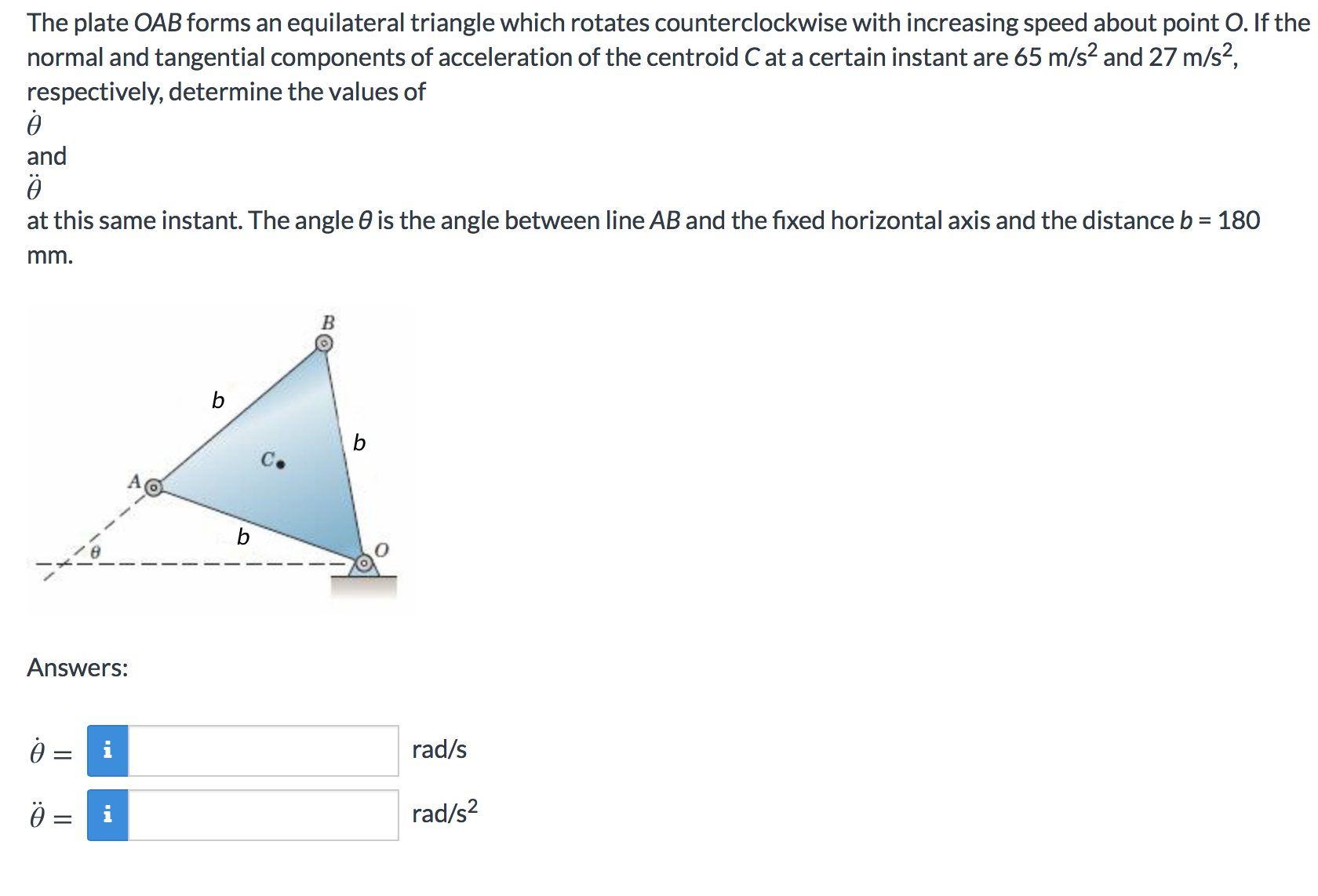# The plate OAB forms an normal and tangential components of acceleration of the centroid C at a certain instant are 65 m/s2 and 27 m/s2, respectively, determine the values of equilateral triangle which rotates counterclockwise with increasing speed about point O. If the and at this same instant. The angle 0 is the angle between line AB and the fixed horizontal axis and the distance b 180 mm. b b b Answers: rad/s rad/s2

Questionhelp_outlineImage TranscriptioncloseThe plate OAB forms an normal and tangential components of acceleration of the centroid C at a certain instant are 65 m/s2 and 27 m/s2, respectively, determine the values of equilateral triangle which rotates counterclockwise with increasing speed about point O. If the and at this same instant. The angle 0 is the angle between line AB and the fixed horizontal axis and the distance b 180 mm. b b b Answers: rad/s rad/s2 fullscreen